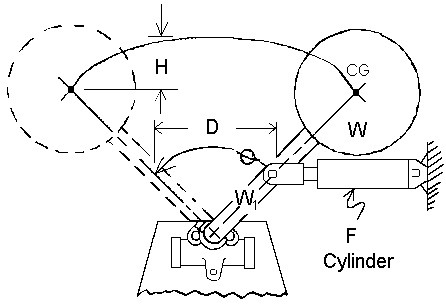RECOMMEND STANDARD SERIES 50Let W = 500#, W1=55#, H=6.7″, D=10″ F=100#, T=955 inch lbs., Ø=1.047 radians.

E = W x H + F x D
E = (500)(6.7) + (100)(10)
E = 4350 IN. LB. ENERGY.

IF THE INPUT POWER IS TORQUE THRU AN ANGLE:

E = W x H + T x Ø
E = (500)(6.7) + (955)(1.047)
E = 4350 IN. LBS. ENERGY

IF THE WEIGHT OF THE ARM (W1) IS GREATER THAN 10% OF W:

E = (W x W1)x H + T x Ø
E = (500 + 55) (6.7) + (955) (1.047)
E = 4718.5 IN. LBS. ENERGY

SYMBOLS

E = Energy                                       Inch Pounds
W = Weight                                     Pounds
V = Contact Velocity                       Ft/Sec.
Note: Contact Engineering Department is contact velocity exceeds 30 ft./sec.
H = Total Vertical Distance           Inches
T = Deceleration Time                   Sec
D = Shock Absorber Stroke           Inches
G = Deceleration Force                  Pounds
F = Driving Force                           Pounds
T = Propelling Torque                    Inch Pounds